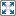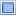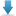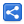[Last Updated: Feb 7, 2017]

`Thread#join()` halts the calling thread execution until the thread represented by this instance terminates.

That means `aThreadInstance.join()` will block the thread in which this method is called until 'aThreadInstance' fishes executing.

Following simple example demonstrates the concept.

```public class ThreadJoinExample {
public static void main (String[] args) throws InterruptedException {
System.out.println("after join");

}

private static class Task implements Runnable {

@Override
public void run () {
int c = 0;
.getName();

for (int i = 0; i < 1000; i++) {
c+=i;
}

}
}
}
```

Output:

```Thread-0 started.
after join
```

### Calculating Factorial of multiple integers in parallel

This is more meaningful example of using join method.

We spawn a single thread A from the main thread which in turn creates n number of threads to calculate factorial of n integers. The threads A waits for all calculator threads to finish before printing the final results.

```public class ThreadJoinExample2 {
public static void main (String[] args) {
final List<Integer> integers = Arrays.asList(10, 12, 13, 14, 15, 20);

@Override
public void run () {
for (Integer integer : integers) {
FactorialCalculator calc = new FactorialCalculator(integer);
calc.start();
}
for (FactorialCalculator calc : threads) {
try {
calc.join();
System.out.println(calc.getNumber() + "! = "
+ calc.getFactorial());

} catch (InterruptedException e) {
e.printStackTrace();
}
}
}
}).start();
}

private static class FactorialCalculator extends Thread {

private final int number;
private BigDecimal factorial;

FactorialCalculator (int number) {
this.number = number;
}

@Override
public void run () {
factorial = calculateFactorial(number);
}

private static BigDecimal calculateFactorial (int number) {
BigDecimal factorial = BigDecimal.ONE;
for (int i = 1; i <= number; i++) {
factorial = factorial.multiply(new BigDecimal(i));
}
return factorial;
}

public BigDecimal getFactorial () {
return factorial;
}

public int getNumber () {
return number;
}
}
}```

Output:

```10! = 3628800
12! = 479001600
13! = 6227020800
14! = 87178291200
15! = 1307674368000
20! = 2432902008176640000
```

• `void join(long millis)`: Waits at most millis milliseconds for this thread to finish. A timeout of 0 means to wait forever.
• `void join(long millis, int nanos) `: Waits at most millis milliseconds plus nanos nanoseconds for this thread to finish.

## Example Project

Dependencies and Technologies Used:

• JDK 1.8
• Maven 3.0.4

 Java Thread Join ExampleSelect AllDownloadShare